Take the Last leap| Aakash BYJU'S JEE (Adv) '23 Mock Test on 28th May Take the Last leap| Aakash BYJU'S JEE (Adv) '23 Mock Test on 28th May

# JEE Main 2022 June 29 – Shift 1 Chemistry Question Paper with Solutions

The JEE Main 2022 June 29 Shift 1 Chemistry question paper with solutions is available here to help students evaluate their performance in the exam. Our subject experts have provided the solutions for all the JEE main 2022 question papers, with simple logic, to obtain the correct answers in less time. You can get accurate solutions and the JEE main answer keys on our website for all the question papers. You can find the solutions for the June 29 Shift 1 chemistry JEE main 2022 question paper on this page.

## JEE Main 2022 June 29th Chemistry Shift 1 Question Paper and Solutions

#### SECTION – A

Multiple Choice Questions: This section contains 20 multiple choice questions. Each question has 4 choices (1), (2), (3) and (4), out of which ONLY ONE is correct.

1. Production of iron in blast furnace follows the following equation

$$\begin{array}{l}Fe_3O_4\left ( s \right )+4CO\left ( g \right )\xrightarrow{~~~~~~~}3Fe\left ( I \right )+4CO_2\left ( g \right )\end{array}$$

when 4.640 kg of Fe3O4 and 2.520 kg of CO are allowed to react then the amount of iron (in g) produced is:

[Given : Molar Atomic mass (g mol–1) : Fe = 56

Molar Atomic mass (g mol–1) : O = 16

Molar Atomic mass (g mol–1) : C = 12]

(A) 1400

(B) 2200

(C) 3360

(D) 4200

Sol.2. Which of the following statements are correct?

(A) The electronic configuration of Cr is [Ar] 3d54s1.

(B) The magnetic quantum number may have a negative value.

(C) In the ground state of an atom, the orbitals are filled in order of their increasing energies.

(D) The total number of nodes are given by n – 2.

Choose the most appropriate answer from the options given below :

(A) (A), (C) and (D) only

(B) (A) and (B) only

(C) (A) and (C) only

(D) (A), (B) and (C) only

Sol. Cr = (Ar)3d54s1

M = +l to –l

As per Aufbau principle, orbitals are filled in increasing order of energy.

Total number of nodes = (n – 1)

3. Arrange the following in the decreasing order of their covalent character :

(A) LiCl

(B) NaCl

(C) KCl

(D) CsCl

Choose the most appropriate answer from the options given below :

(A) (A) > (C) > (B) > (D)

(B) (B) > (A) > (C) > (D)

(C) (A) > (B) > (C) > (D)

(D) (A) > (B) > (D) > (C)

Sol. Covalent character ∝ polarising power of cation

Correct decreasing order of covalent character

LiCl > NaCl > KCl > CsCl

4. The solubility of AgCl will be maximum in which of the following?

(A) 0.01 M KCl

(B) 0.01 M HCl

(C) 0.01 M AgNO3

(D) Deionised water

Sol. Solubility decreases with increasing the concentration of common ion. Therefore, the maximum solubility of AgCl will be in deionized water.

5. Which of the following is a correct statement?

(A) Brownian motion destabilises sols.

(B) Any amount of dispersed phase can be added to emulsion without destabilising it.

(C) Mixing two oppositely charged sols in equal amount neutralises charges and stabilises colloids.

(D) Presence of equal and similar charges on colloidal particles provides stability to the colloidal solution.

Sol. Presence of equal and similar charges on colloidal particle provides stability to the colloidal solution.

6. The electronic configuration of Pt(atomic number 78) is:

(A) [Xe] 4f14 5d9 6s1

(B) [Kr] 4f14 5d10

(C) [Xe] 4f14 5d10

(D) [Xe] 4f14 5d8 6s2

Sol. Pt = [Xe] 4f14 5d9 6s1

7. In isolation of which one of the following metals from their ores, the use of cyanide salt is not commonly involved?

(A) Zinc

(B) Gold

(C) Silver

(D) Copper

Sol. In the extraction of Silver and Gold, NaCN is used to leach the metal.

$$\begin{array}{l}4Au\left ( s \right )+8C\overline{N}\left ( aq \right )+2H_2O+O_2\left ( g \right )\rightarrow 4\left [ Au\;\left ( CN \right )_2 \right ]^-\left ( aq \right )+4OH^-\left ( aq \right )\end{array}$$
$$\begin{array}{l}2\left [ Au\left ( CN \right )_2 \right ]^-\left ( aq \right )+Zn\rightarrow 2Au\left ( s\right )+\left [ \left ( Zn\left ( CN \right )_4 \right ) \right ]^{2-}\left ( aq \right )\end{array}$$

In case of ore containing ZnS and PbS, the depressant used is NaCN.

8. Which one of the following reactions indicates the reducing ability of hydrogen peroxide in basic medium?

(A) HOCI + H2O2 → H3O+ + CI+ O2

(B) PbS + 4H2O2 → PbSO4 + 4H2O

$$\begin{array}{l}(C)\ 2MnO_4^-+3H_2O_2\rightarrow2MnO_2+3O_2+2H_2O+2OH^-\end{array}$$
$$\begin{array}{l}(D)\ Mn^{2+}+H_2O_2\rightarrow Mn^{4+}+2OH^-\end{array}$$

Sol.

$$\begin{array}{l}2Mn\overset{+7}{O_4^-}+3H_2O_2\rightarrow \overset{+4}{2MnO_2}+3O_2+2H_2O+2O\overset{-}{H}\end{array}$$

In basic medium MnO4 is reduced to MnO2, whereas in acidic medium it is reduced to Mn+2.

9. Match List-I with List-II.

 List-I (Metal) List-II (Emitted light wavelength (nm)) (A) Li (I) 670.8 (B) Na (II) 589.2 (C) Rb (III) 780.0 (D) Cs (IV) 455.5

Choose the most appropriate answer from the options given below:

(A) (A)-(I), (B)-(II), (C)-(III), (D)-(IV)

(B) (A)-(III), (B)-(II), (C)-(I), (D)-(IV)

(C) (A)-(III), (B)-(I), (C)-(II), (D)-(IV)

(D) (A)-(IV), (B)-(II), (C)-(I), (D)-(III)

Sol.

 Metal Li Na K Rb Cs Colour Crimson-red Yellow Violet Red violet Blue λ/nm 670.8 589.2 766.5 780.0 455.5

10. Match List-I with List-II.

 List-I (Metal) List-II (Application) (A) Cs (I) High temperature thermometer (B) Ga (II) Water repellent sprays (C) B (III) Photoelectric cells (D) Si (IV) Bullet proof vest

Choose the most appropriate answer from the options given below:

(A) (A)-(III), (B)-(I), (C)-(IV), (D)-(II)

(B) (A)-(IV), (B)-(III), (C)-(II), (D)-(I)

(C) (A)-(II), (B)-(III), (C)-(IV), (D)-(I)

(D) (A)-(I), (B)-(IV), (C)-(II), (D)-(III)

Sol. Metal Application

Cs Photoelectric cells

Ga High temperature thermometer

B Bullet proof vest

Si Water repellent sprays

11. The oxoacid of phosphorus that is easily obtained from a reaction of alkali and white phosphorus and has two P-H bonds, is:

(A) Phosphonic acid

(B) Phosphinic acid

(C) Pyrophosphorus acid

(D) Hypophosphoric acid

Sol. White phosphorus + alkali H3PO2

H3PO2 = phosphinic acid

12. The acid that is believed to be mainly responsible for the damage of Taj Mahal is

(A) sulfuric acid

(B) hydrofluoric acid

(C) phosphoric acid

(D) hydrochloric acid

Sol. CaCO3 + H2SO4 → CaSO4 + H2O + CO2

13. Two isomers ‘A’ and ‘B’ with molecular formula C4H8 give different products on oxidation with KMnO4/H+ results in effervescence of a gas and gives ketone. The compound ‘A’ is

(A) But-1-ene

(B) cis-But-2-ene

(C) trans-But-2-ene

(D) 2-methyl propene

Sol.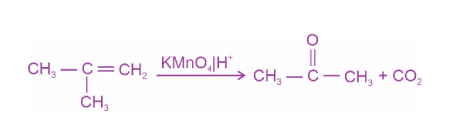14.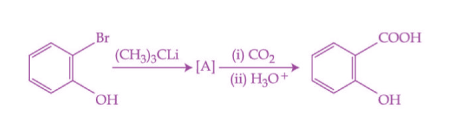In the given conversion the compound A is: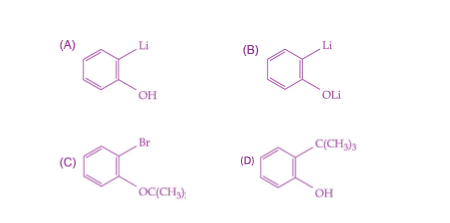Sol.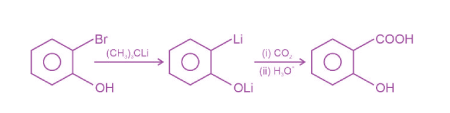15. Given below are two statements:

Statement I: The esterification of carboxylic acid with an alcohol is a nucleophilic acyl substitution.

Statement II: Electron withdrawing groups in the carboxylic acid will increase the rate of esterification reaction.

Choose the most appropriate option :

(A) Both Statement I and Statement II are correct.

(B) Both Statement I and Statement II are incorrect.

(C) Statement I is correct but Statement II is incorrect.

(D) Statement I is incorrect but Statement II is correct.

Sol. Esterification of carboxylic acid with an alcohol is nucleophilic acyl substitution and presence of electron withdrawing group in the carboxylic acid increases the rate of esterification reaction.

16.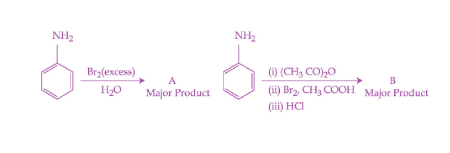Consider the above reactions, the product A and product B, respectively are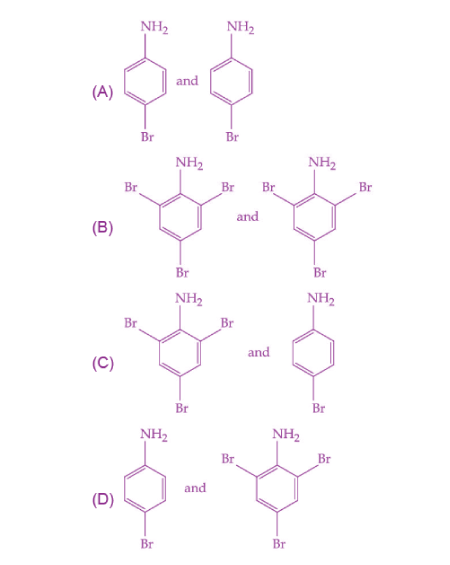Sol.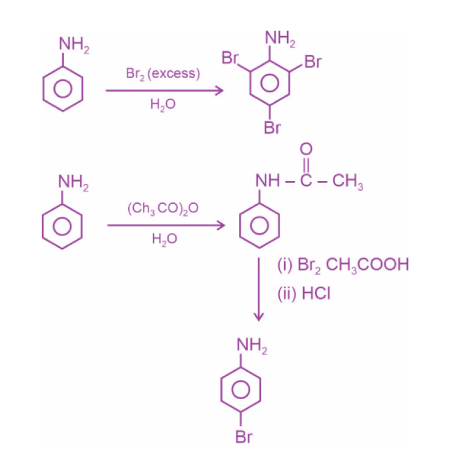17. The polymer, which can be stretched and retains its original status on releasing the force is

(A) Bakelite

(B) Nylon 6, 6

(C) Buna-N

(D) Terylene

Sol. Buna – N is a synthetic rubber which can be stretched and retains its original status on releasing the force.

18. Sugar moiety in DNA and RNA molecules, respectively are

(A) β-D-2-deoxyribose, β-D-deoxyribose

(B) β-D-2-deoxyribose, β-D-ribose

(C) β-D-ribose, β-D-2-deoxyribose

(D) β-D-deoxyribose, β-D-2-deoxyribose

Sol. DNA consists of β-D-2-deoxyribose sugar whereas RNA consists of β-D-ribose.

19. Which of the following compound does not contain sulphur atom?

(A) Cimetidine

(B) Ranitidine

(C) Histamine

(D) Saccharin

Sol. Histamine – does not contain sulphur.20. Given below are two statements.

Statement I : Phenols are weakly acidic.

Statement II : Therefore they are freely soluble in NaOH solution and are weaker acids than alcohols and water.

Choose the most appropriate option.

(A) Both Statement I and Statement II are correct.

(B) Both Statement I and Statement II are correct.

(C) Statement I is correct but Statement II is incorrect.

(D) Statement I is incorrect but Statement II is correct.

Sol.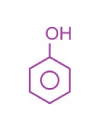pKa = 10

Alcohol pKa ≈ 16

H2O pKa ≈ 15

#### SECTION – B

Numerical Value Type Questions: This section contains 10 questions. In Section B, attempt any five questions out of 10. The answer to each question is a NUMERICAL VALUE. For each question, enter the correct numerical value (in decimal notation, truncated/rounded-off to the second decimal place; e.g. 06.25, 07.00, –00.33, –00.30, 30.27, –27.30) using the mouse and the on-screen virtual numeric keypad in the place designated to enter the answer.

1. Geraniol, a volatile organic compound, is a component of rose oil. The density of the vapour is 0.46 gL–1 at 257°C and 100 mm Hg. The molar mass of geraniol is ____ g mol –1. (Nearest Integer) [Given: R = 0.082 L atm K–1 mol–1]

Sol.

$$\begin{array}{l}\frac{PM}{RT}=d=M=\frac{0.46\times0.082\times530\times760}{100}\end{array}$$

≃ 152 g mol–1

2. 17.0 g of NH3 completely vapourises at – 33.42°C and 1 bar pressure and the enthalpy change in the process is 23.4 kJ mol–1 . The enthalpy change for the vapourisation of 85 g of NH3 under the same conditions is ____ kJ.

Sol. Number of moles of NH3 = 5

So, required ΔH = 5 × 23.4

= 117 kJ

3. 1.2 mL of acetic acid is dissolved in water to make 2.0 L of solution. The depression in freezing point observed for this strength of acid is 0.0198°C. The percentage of dissociation of the acid is ____ . (Nearest integer)

[Given: Density of acetic acid is 1.02 g mL–1

Molar mass of acetic acid is 60 g mol–1

Kf(H2O) = 1.85 K kg mol–1]

Sol. ΔTb = i × Kb × m

Moles of solute (acetic acid)

$$\begin{array}{l}=\frac{1.2\times1.02}{60} \end{array}$$

As moles of solute are very less.

So, take molarity and molality same.

$$\begin{array}{l}0.0198 = i\times 1.85 \times \frac{1.2\times1.02}{60\times2}\end{array}$$

i = 1.05

$$\begin{array}{l}\alpha=\frac{i-1}{n-1}=\frac{0.05}{1}=0.05 \end{array}$$

4. A dilute solution of sulphuric acid is electrolysed using a current of 0.10 A for 2 hours to produce hydrogen and oxygen gas. The total volume of gases produced at STP is ____ cm3. (Nearest integer)

[Given : Faraday constant F = 96500 C mol–1 at STP, molar volume of an ideal gas is 22.7 L mol–1]

Sol. 2 F produces = 3/2 mole of gas

0.10 ×2 × 3600 coulomb produces

$$\begin{array}{l}=\frac{\frac{3}{2}\times0.1\times2\times3600}{2\times96500}\end{array}$$

= 0.0056 moles of gas

Volume of gas produced = 0.0056 × 22.7 L

≃ 0.127 L

= 127 mL

5. The activation energy of one of the reactions in a biochemical process is 532611 J mol–1. When the temperature falls from 310 K to 300 K, the change in rate constant observed is k300 = x × 10–3 k310. The value of x is ______.

[Given: ln10 = 2.3, R = 8.3 JK–1 mol–1]

Sol.

$$\begin{array}{l}log\left ( \frac{K_{310}}{K_{300}} \right )=\frac{532611}{8.3}\left ( \frac{1}{300}-\frac{1}{310} \right )\end{array}$$
$$\begin{array}{l}\frac{K_{310}}{K_{300}}=10^3~~\Rightarrow~~K_{300}=1\times10^{-3}\times K_{310}\end{array}$$

6. The number of terminal oxygen atoms present in the product B obtained from the following reaction is ________.

FeCr2O4 + Na2CO3 + O2 → A + Fe2O3 + CO2

A + H+ → B + H2O + Na+

Sol.

$$\begin{array}{l}FeCr_2O_4+Na_2CO_3+O_2\rightarrow{~~~~~~~}Fe_2O_3+CO_2+\underset{\left ( A \right )}{Na_2CrO_4} \end{array}$$
$$\begin{array}{l}Na_2CrO_4+H^+\rightarrow{~~~~~~~}Cr_2O_7^{2-}+H_2O+Na^+\end{array}$$7. An acidified manganate solution undergoes disproportionation reaction. The spin-only magnetic moment value of the product having manganese in higher oxidation state is ____ B.M. (Nearest integer)

Sol.

$$\begin{array}{l}MnO_4^{2-}\xrightarrow{~~~H^+~~~}MnO_2+MnO_4^-+H_2O \end{array}$$

Mn+7 = d0

Hence, magnetic moment = zero

8. Kjeldahl’s method was used for the estimation of nitrogen in an organic compound. The ammonia evolved from 0.55 g of the compound neutralised 12.5 mL of 1 M H2SO4 solution. The percentage of nitrogen in the compound is _____. (Nearest integer)

Sol.

$$\begin{array}{l}\% N = \frac{1.4\times N\times V}{\textup{Mass of organic compound}}\end{array}$$
$$\begin{array}{l}=\frac{1.4\times2\times12.5}{0.55}=63.63\%\simeq64\end{array}$$

9. Observe structures of the following compounds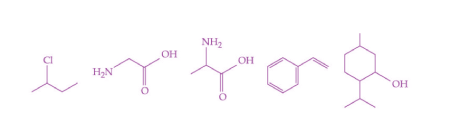The total number of structures/compounds which possess asymmetric carbon atoms is _____.

Sol.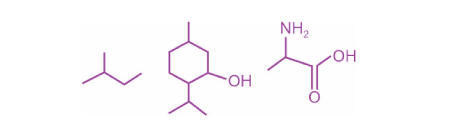10.

$$\begin{array}{l}C_6H_{12}O_6\xrightarrow{~~Zymase~~}A\xrightarrow[\Delta]{NaOI}B+CHl_3\end{array}$$

The number of carbon atoms present in the product B is ______.

Sol.

$$\begin{array}{l}C_6H_{12}O_6\xrightarrow{~~\textup{zymase}~~}C_2H_5OH\xrightarrow{NaOl}\underset{\left ( B \right )}{HCOONa}+CHl_3\end{array}$$

### Download PDF of JEE Main 2022 June 29 Shift 1 Chemistry Paper & Solutions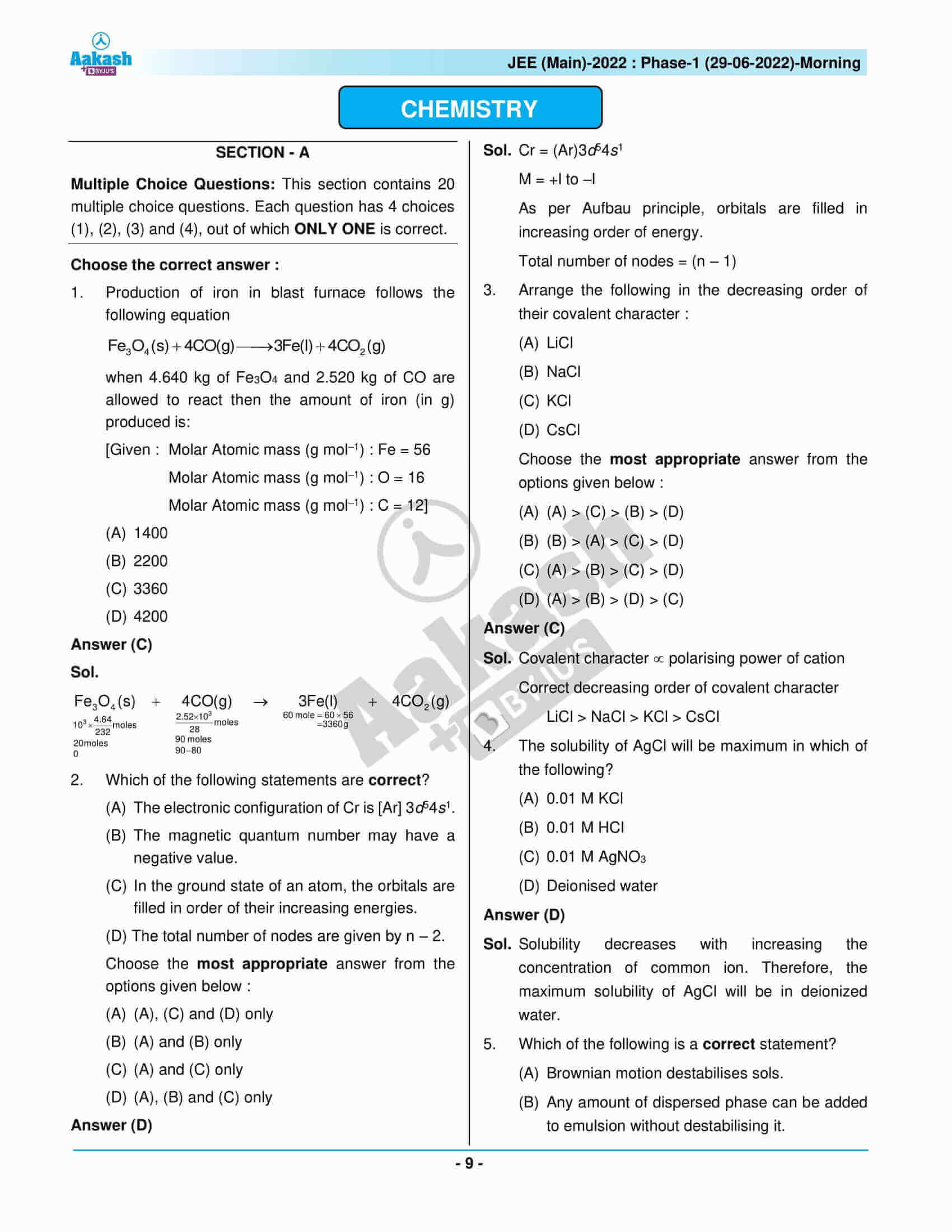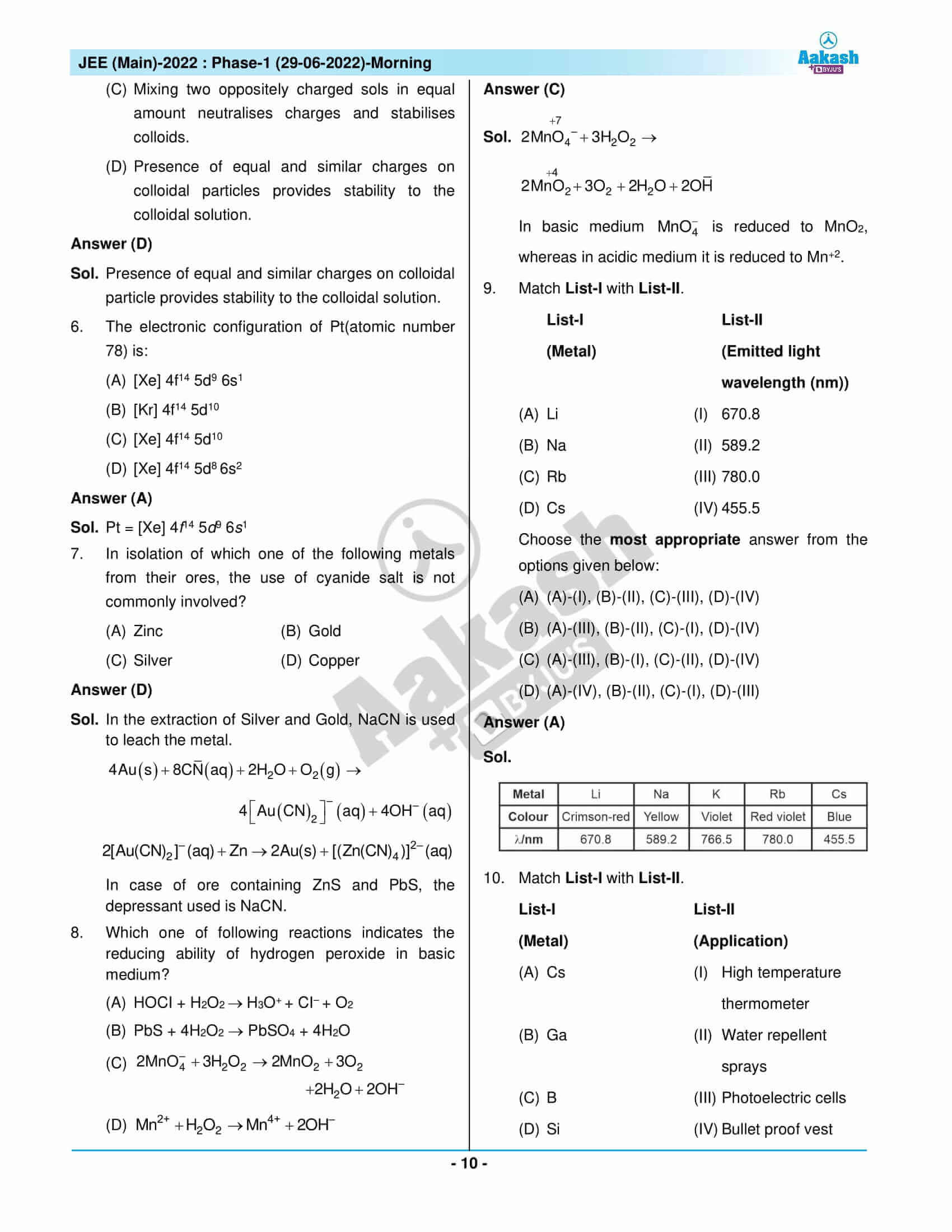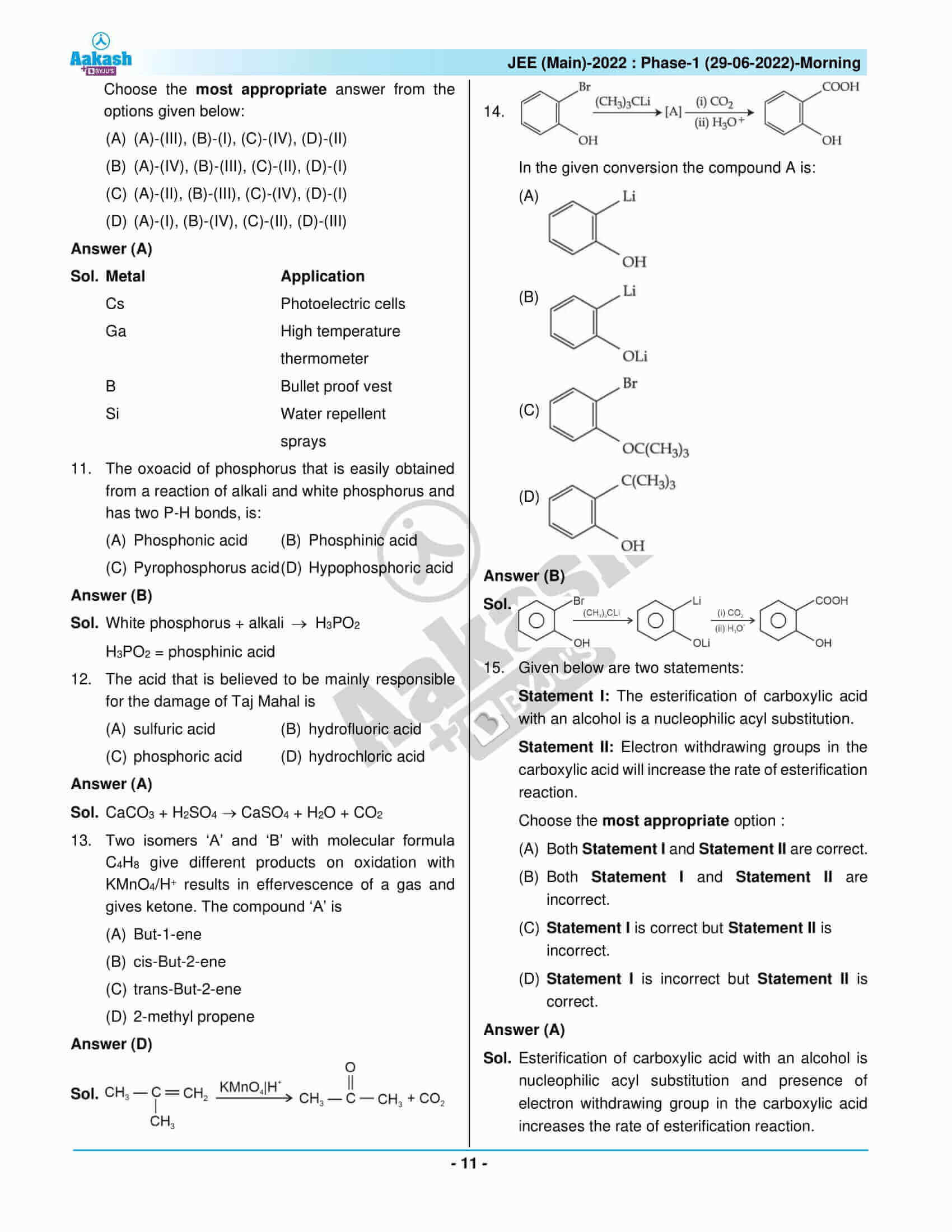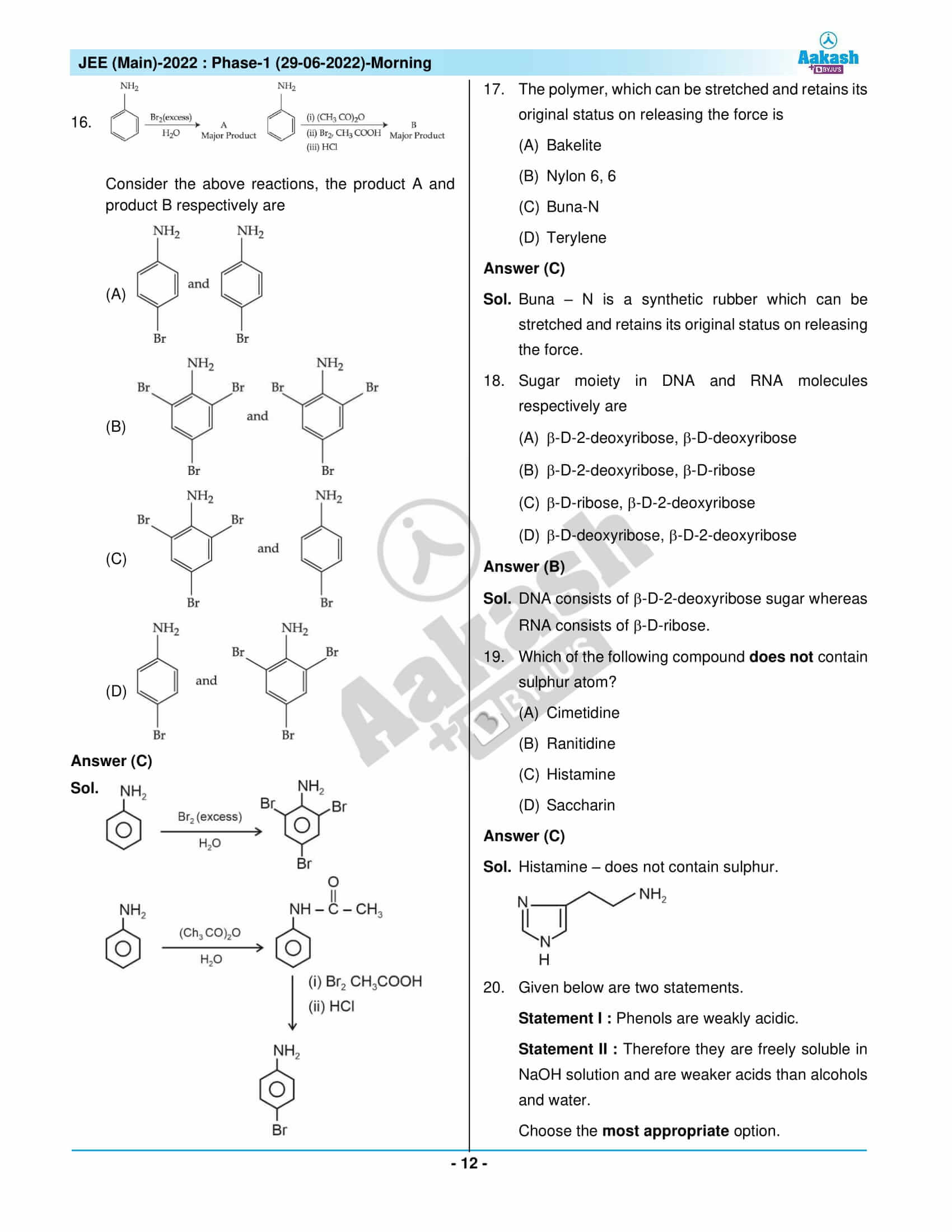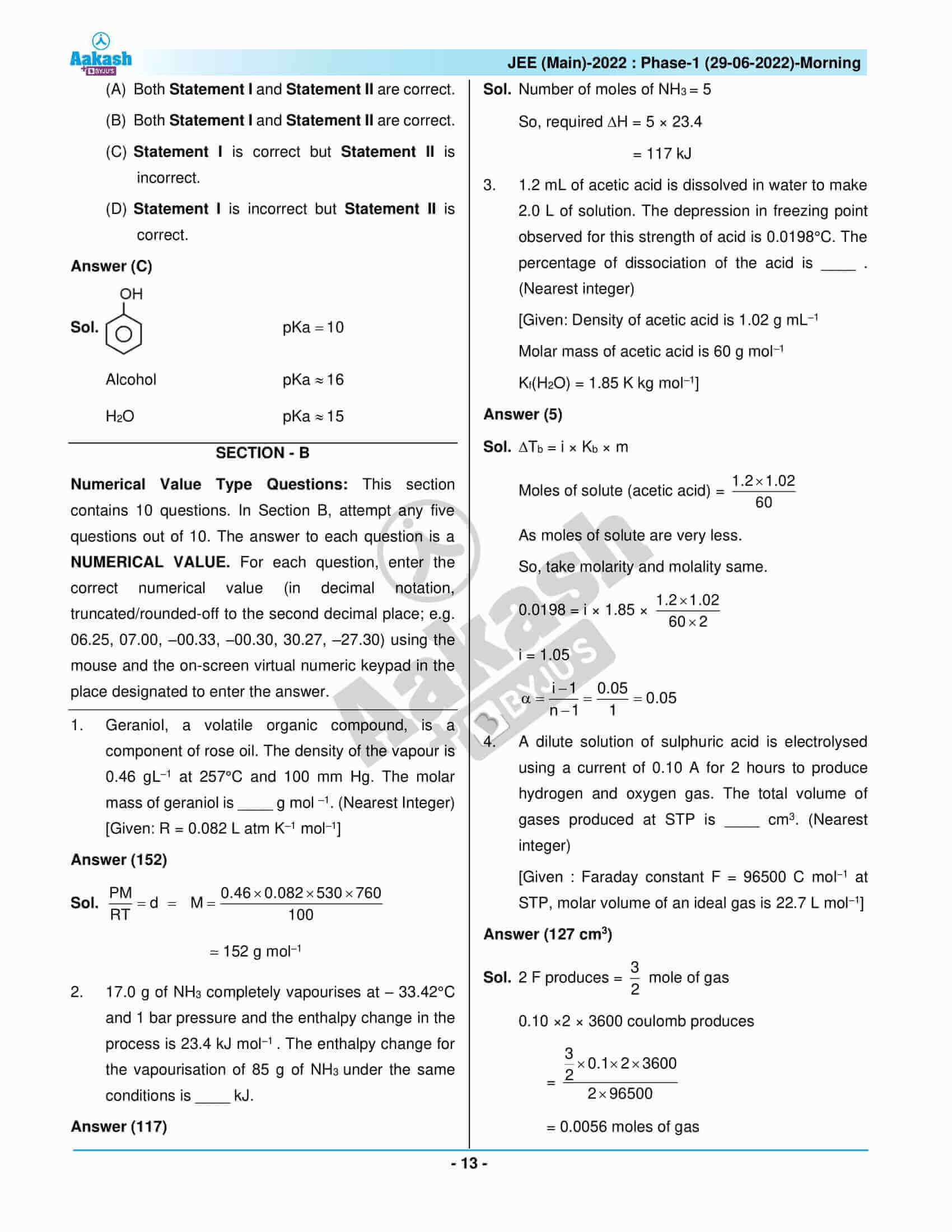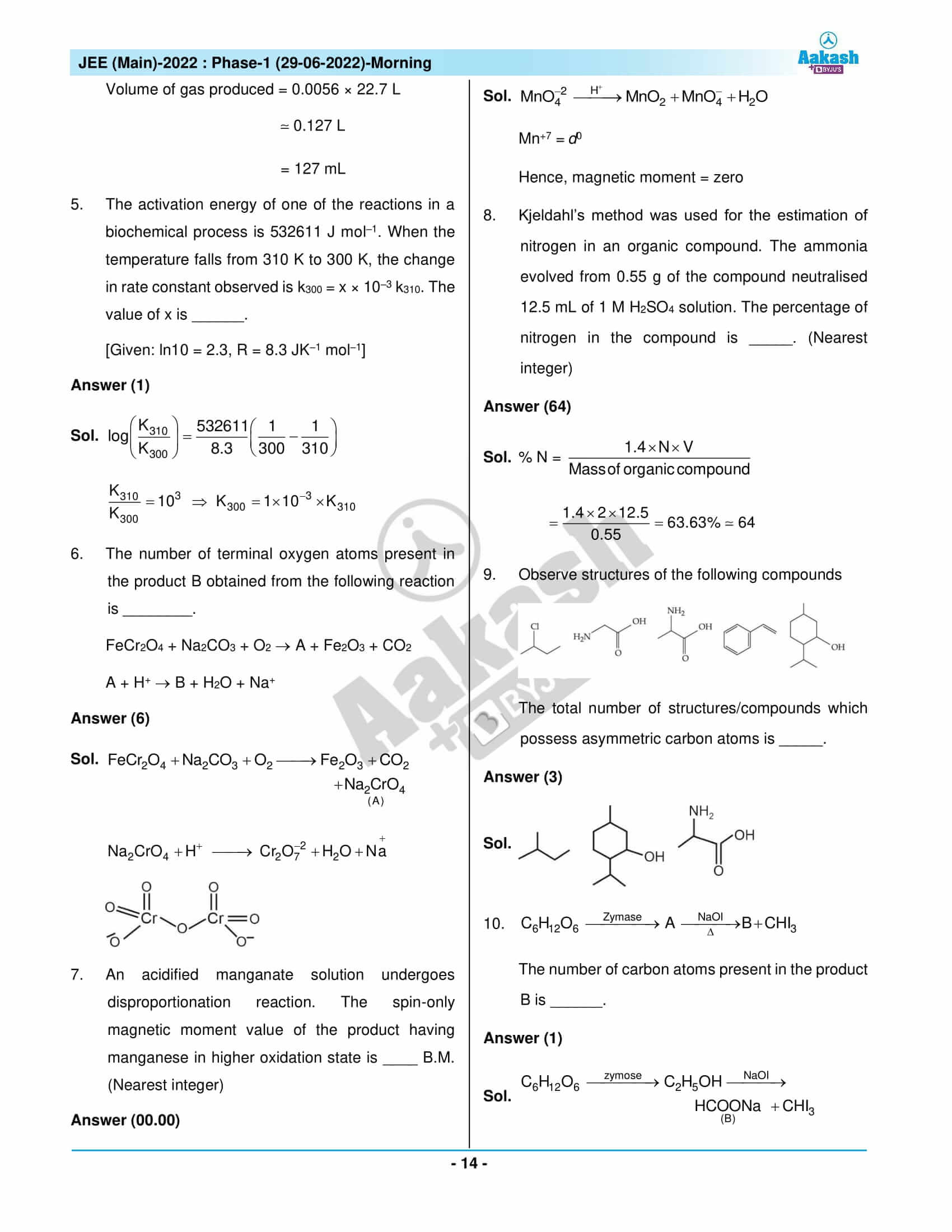## JEE Main 2022 June 29 Shift 1 Paper with Solutions

### JEE Main 2022 June 29 Shift 1 Question Paper – Physics Solutions### JEE Main 2022 June 29 Shift 1 Question Paper – Chemistry Solutions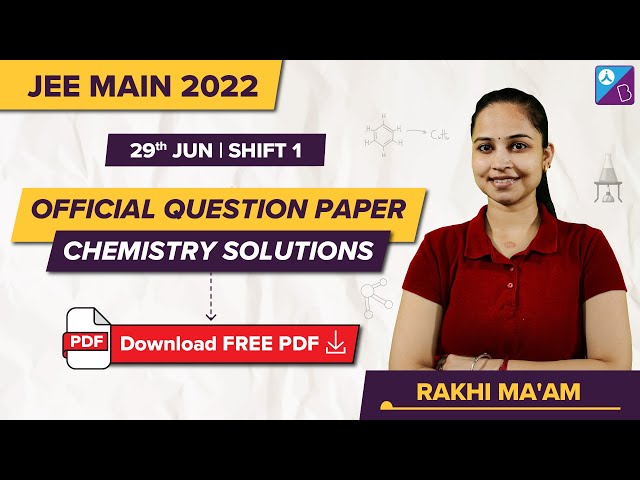### JEE Main 2022 June 29 Shift 1 Question Paper – Maths Solutions## Frequently Asked Questions – FAQs

Q1

### How do you rate the difficulty level of the JEE Main 2022 June 29 Chemistry Shift 1 question paper?

We can say that the difficulty level of the JEE Main 2022 June 29 Chemistry Shift 1 question paper was 1.43 out of 3.

Q2

### How many questions are there in the JEE Main 2022 Chemistry June 29 Shift 1 question paper?

There are 30 questions in the JEE Main 2022 Chemistry June 29 Shift 1 question paper.

Q3

### What is the total mark of the JEE Main 2022 Shift 1 Chemistry June 29 question paper?

The total mark of the JEE Main 2022 Shift 1 Chemistry June 29 question paper is 120.

Q4

### Which topic was covered in the chapter Environmental Chemistry for the JEE Main 2022 June 29 Chemistry Shift 1 question paper?

The topic covered in the chapter Environmental Chemistry was atmospheric pollution.

Q5

### What is the difficulty level of questions asked from States of matter in JEE Main 2022 June 29 Shift 1 Chemistry question paper?

The questions asked from States of matter in JEE Main 2022 June 29 Shift 1 Chemistry question paper were easy.